# 模拟退火算法

minxXf(x). min x ∈ X f ( x ) .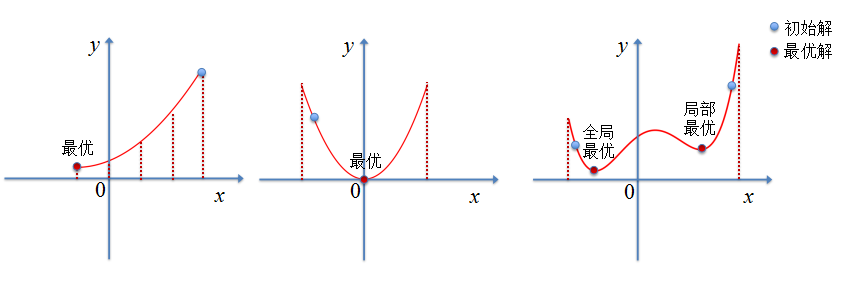### 一、模拟退火算法基本思想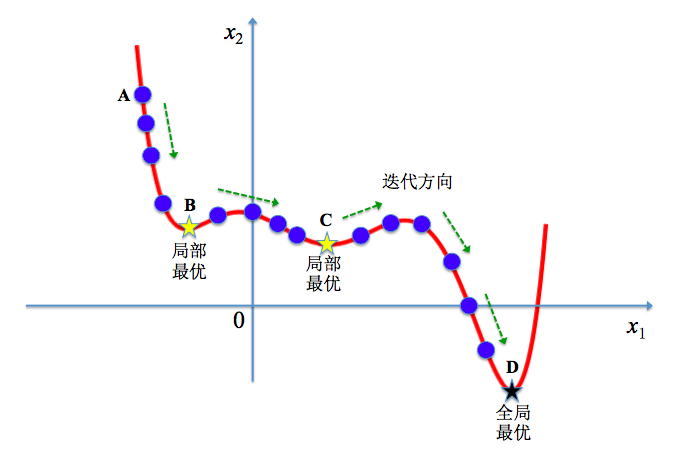p(dE)=exp(dEkT). p ( d E ) = exp ⁡ ( d E k T ) .

Δf=f(x_new)f(x). Δ f = f ( x _ n e w ) − f ( x ) .

p(Δf)=exp(ΔfkT)exp(ΔfkT) p ( Δ f ) = { exp ⁡ ( − Δ f k T ) 最 小 值 优 化 问 题 exp ⁡ ( Δ f k T ) 最 大 值 优 化 问 题

### 二、模拟退火算法描述

1. 初始化：初始温度 T T $T$（充分大），温度下限${T}_{min}$$T_{min}$（充分小），初始解状态 x x $x$(是算法迭代的起点)，每个$T$$T$值的迭代次数 L L $L$
2. $l=1,2,...,L$$l= 1,2,...,L$做第3至第6步；
3. 产生新解 x_new x _ n e w $x\_new$: ( x_new=x+Δx x _ n e w = x + Δ x $x\_new = x + Δx$)；
4. 利计算增量 Δf=f(x_new)f(x) Δ f = f ( x _ n e w ) − f ( x ) $Δf = f(x\_new) − f(x)$，其中 f(x) f ( x ) $f(x)$为优化目标；
5. Δf<0 Δ f < 0 $Δf<0$(若寻找最大值， Δf>0 Δ f > 0 $Δf>0$)则接受 x_new x _ n e w $x\_new$作为新的当前解，否则以概率 exp(Δf/(kT)) exp ⁡ ( − Δ f / ( k T ) ) $\exp(-Δf/(kT ))$接受 x_new x _ n e w $x\_new$作为新的当前解；
6. 如果满足终止条件则输出当前解作为最优解，结束程序。(终止条件通常取为连续若干个新解都没有被接受时终止算法。)；
7. T T $T$逐渐减少，且$T>{T}_{min}$$T> T_{min}$，然后转第2步。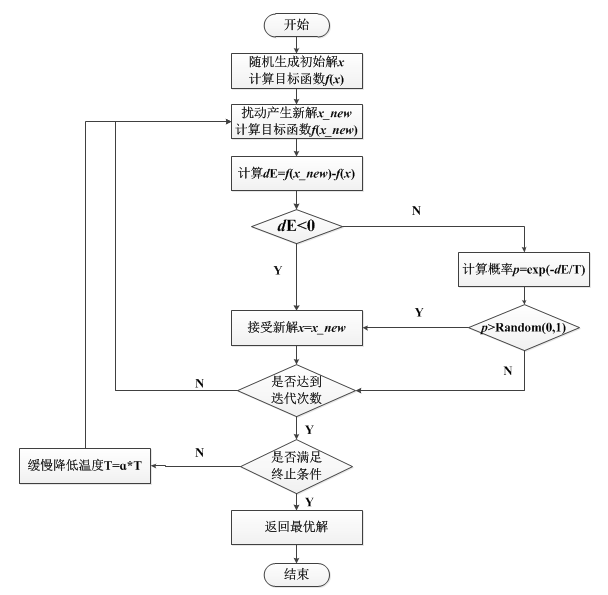### 三、模拟退火算法的优缺点

(1) 温度T的初始值设置问题
温度 T T $T$的初始值设置是影响模拟退火算法全局搜索性能的重要因素之一、初始温度高，则搜索到全局最优解的可能性大，但因此要花费大量的计算时间；反之，则可节约计算时间，但全局搜索性能可能受到影响。

(2) 退火速度问题，即每个$T$$T$值的迭代次数
模拟退火算法的全局搜索性能也与退火速度密切相关。一般来说，同一温度下的“充分”搜索是相当必要的，但这也需要计算时间。循环次数增加必定带来计算开销的增大。

(3) 温度管理问题
温度管理问题也是模拟退火算法难以处理的问题之一。实际应用中，由于必须考虑计算复杂度的切实可行性等问题，常采用如下所示的降温方式：

T=α×T.α(0,1). T = α × T . α ∈ ( 0 , 1 ) .

### 四、模拟退火算法Python实战

minf(x)=(x2)(x+3)(x+8)(x9) min f ( x ) = ( x − 2 ) ∗ ( x + 3 ) ∗ ( x + 8 ) ∗ ( x − 9 )

# -*- coding: utf-8 -*-
import numpy as np
import matplotlib.pyplot as plt

def inputfun(x):
return (x-2)*(x+3)*(x+8)*(x-9)

initT = 1000 #初始温度
minT = 1 #温度下限
iterL = 1000 #每个T值的迭代次数
delta = 0.95 #温度衰减系数
k = 1

initx = 10*(2*np.random.rand()-1)
nowt = initT
print "初始解：",initx

xx = np.linspace(-10,10,300)
yy = inputfun(xx)
plt.figure()
plt.plot(xx,yy)
plt.plot(initx,inputfun(initx),'o')

#模拟退火算法寻找最小值过程
while nowt>minT:
for i in np.arange(1,iterL,1):
funVal = inputfun(initx)
xnew = initx+(2*np.random.rand()-1)
if xnew>=-10 and xnew<=10:
funnew = inputfun(xnew)
res = funnew-funVal
if res<0:
initx = xnew
else:
p = np.exp(-(res)/(k*nowt))
if np.random.rand()<p:
initx = xnew
#            print initx-xnew
#    print initx
#    print nowt
nowt = nowt*delta

print "最优解：",initx
print "最优值：",inputfun(initx)
plt.plot(initx,inputfun(initx),'*r')
plt.show()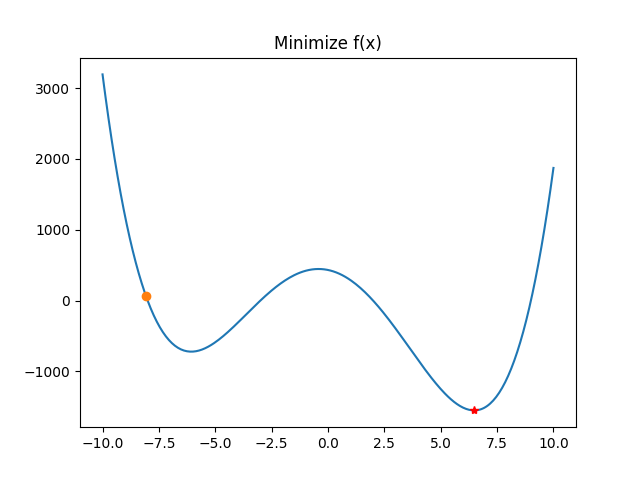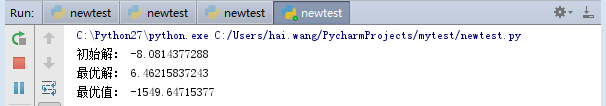### 参考资料

1. http://wiki.mbalib.com/wiki/模拟退火算法 模拟退火算法
05-22123
02-266884

12-241943
10-194453
10-252万+
03-11859
02-117779
04-307万+
11-1615万+
12-18277
03-031288
03-041059
04-07996
09-239396
04-121319
06-103994

### “相关推荐”对你有帮助么？

•非常没帮助
•没帮助
•一般
•有帮助
•非常有帮助AI_BigData_WH

¥2 ¥4 ¥6 ¥10 ¥20余额支付 (余额：-- )扫码支付获取中扫码支付点击重新获取扫码支付1.余额是钱包充值的虚拟货币，按照1:1的比例进行支付金额的抵扣。
2.余额无法直接购买下载，可以购买VIP、C币套餐、付费专栏及课程。余额充值